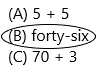# Texas Go Math Grade 2 Lesson 1.1 Answer Key Different Ways to Represent Numbers

Refer to our Texas Go Math Grade 2 Answer Key Pdf to score good marks in the exams. Test yourself by practicing the problems from Texas Go Math Grade 2 Lesson 1.1 Answer Key Different Ways to Represent Numbers.

## Texas Go Math Grade 2 Lesson 1.1 Answer Key Different Ways to Represent Numbers

Explore

Write the number. Then write it another way.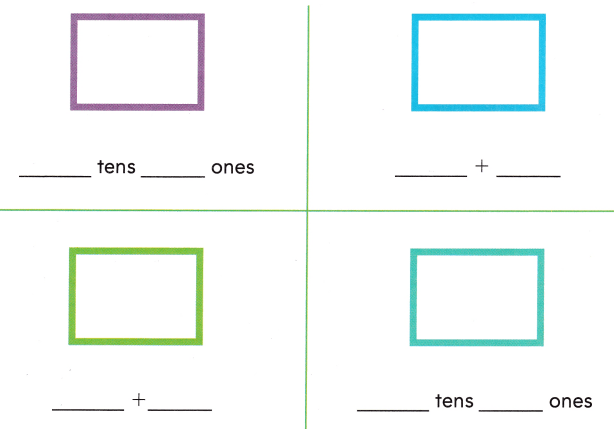FOR THE TEACHER • Read the following problem. Taryn counted 53 books on the shelves. How many tens and ones are in 53? Continue the activity with the numbers 78, 35, and 40.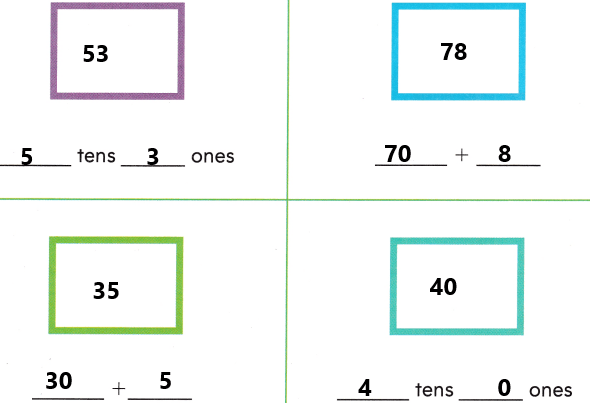Math Talk
Mathematical Processes

In 44, do both digits have the same value? Explain.
Explanation:
The place values will change definitely.
44 can be written as 40+4.
The number 44, 4 is in the tens place and another 4 is in one’s place.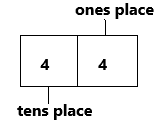Model and Draw

You can write a number in different ways.
What is this number?
fifty-nine
50 + 9Share and Show

Look at the examples above. Write the number. Then write the number another way.

Question 1.
thirty-two
The expanded form:
The expanded form of the numbers helps to determine the place value of each digit in the given number. It means that the expansion of numbers is based on the place value. The expanded form splits the number, and it represents the number in units, tens, hundreds and thousands form.
The expanded form of 32 is 30+2. In the number 32, the number 3 is in the tens place and 2 is in one’s place.

Question 2.
20 + 7
20+7=27
Explanation:
Word form: Word form is writing the numerical/number as you would say it in words. Word form is one way in which the concept of numbers can be written. Other forms include standard and expanded forms. Word form involves expressing numbers using words rather than numerals.
The word form of 27 is twenty-seven.

Question 3.
60 + 3
60+3 is nothing but 63.
We can write it in another way which is in word form.
Word form: Word form is writing the numerical/number as you would say it in words. Word form is one way in which the concept of numbers can be written. Other forms include standard and expanded forms. Word form involves expressing numbers using words rather than numerals.
The word form of 63 is sixty-three.

Question 4
ninety-five
The word form ninety-five can be written in number 95.
In another way we can write is in expanded form:
The expanded form of the numbers helps to determine the place value of each digit in the given number. It means that the expansion of numbers is based on the place value. The expanded form splits the number, and it represents the number in units, tens, hundreds and thousands form.
The expanded form of 95 is 90+5. In the number 95, the number 9 is in the tens place and 5 is in one’s place.

Question 5.
50 + 1
The expanded form 50+1 can be written in number 51.
In another way, we can write in word form.
Word form: Word form is writing the numerical/number as you would say it in words. Word form is one way in which the concept of numbers can be written. Other forms include standard and expanded forms. Word form involves expressing numbers using words rather than numerals.
The word form of 51 is fifty-one.

Question 6.
seventy-six
The word form seventy-six can be written in number 76.
In another way we can write is in expanded form:
The expanded form of the numbers helps to determine the place value of each digit in the given number. It means that the expansion of numbers is based on the place value. The expanded form splits the number, and it represents the number in units, tens, hundreds and thousands form.
The expanded form of 76 is 70+6. In the number 76, the number 7 is in the tens place and 6 is in one’s place.

Question 7.
twenty-eight
The word form twenty-eight can be written in number 28.
In another way we can write is in expanded form:
The expanded form of the numbers helps to determine the place value of each digit in the given number. It means that the expansion of numbers is based on the place value. The expanded form splits the number, and it represents the number in units, tens, hundreds and thousands form.
The expanded form of 28 is 20+8. In the number 28, the number 2 is in the tens place and 8 is in one’s place.

Question 8.
80 + 0
The expanded form 80+0 can be written in number 80.
In another way, we can write in word form.
Word form: Word form is writing the numerical/number as you would say it in words. Word form is one way in which the concept of numbers can be written. Other forms include standard and expanded forms. Word form involves expressing numbers using words rather than numerals.
The word form of 80 is eighty.

Problem Solving

Write the number another way.

Question 9.
20 + 4
Explanation:
We can write in word form, standard form, pictorial representation, and place value chart. Moreover, it’s already given in the expanded form.
Standard form:
The standard form of 24 is 2.4×10^1.
1. First we need to place a decimal point then it will become 2.4
2. Count the digits after the decimal place.
– There is one digit after the decimal.
3. Raise this number to the power of 10 and multiply it to the original value.
– 2.4×10^1
Word form: Word form is writing the numerical/number as you would say it in words. Word form is one way in which the concept of numbers can be written. Other forms include standard and expanded forms. Word form involves expressing numbers using words rather than numerals.
The word form of 24 is twenty-four.
Question 10.
37
Explanation:
We can write 37 in expanded form and word form.
Expanded form: The expanded form of the numbers helps to determine the place value of each digit in the given number. It means that the expansion of numbers is based on the place value. The expanded form splits the number, and it represents the number in units, tens, hundreds and thousands form. Therefore, the expanded form of 37 is 30+7. In the number 37, the number 3 is in the tens place and 7 is in one’s place.
Word form: Word form is writing the numerical/number as you would say it in words. Word form is one way in which the concept of numbers can be written. Other forms include standard and expanded forms. Word form involves expressing numbers using words rather than numerals. The word form for 2,010 is two thousand ten. The word form for 1,000,000 is one million. Likewise, 37 is given so it can be written as thirty-seven.

Question 11.
eighty-five
We can write eighty-five in expanded form and standard form.
Expanded form: The expanded form of the numbers helps to determine the place value of each digit in the given number. It means that the expansion of numbers is based on the place value. The expanded form splits the number, and it represents the number in units, tens, hundreds and thousands form. The expanded form of 85 is 80+5. In the number 85, the number 8 is in the tens place and 5 is in one’s place.
Standard form:
The standard form of 85 is 8.5×10^1.
1. First we need to place a decimal point then it will become 8.5
2. Count the digits after the decimal place.
– There is one digit after the decimal.
3. Raise this number to the power of 10 and multiply it to the original value.
– 8.5×10^1

Question 12.
54
Explanation:
We can write this in expanded form and word form.
Expanded form: The expanded form of the numbers helps to determine the place value of each digit in the given number. It means that the expansion of numbers is based on the place value. The expanded form splits the number, and it represents the number in units, tens, hundreds and thousands form. The expanded form of 54 is 50+4. In the number 54, the number 5 is in the tens place and 4 is in one’s place.
Word form: Word form is writing the numerical/number as you would say it in words. Word form is one way in which the concept of numbers can be written. Other forms include standard and expanded forms. Word form involves expressing numbers using words rather than numerals. The word form for 2,010 is two thousand ten. The word form for 1,000,000 is one million. Likewise, 54 is given so it can be written as fifty-four.

Question 13.
twelve
Explanation:
We can write twelve in expanded form and standard form.
Expanded form: The expanded form of the numbers helps to determine the place value of each digit in the given number. It means that the expansion of numbers is based on the place value. The expanded form splits the number, and it represents the number in units, tens, hundreds and thousands form. The expanded form of 12 is 10+2. In the number 12, the number 1 is in the tens place and 2 is in one’s place.
Standard form:
The standard form of 12 is 1.2×10^1.
1. First we need to place a decimal point then it will become 1.2
2. Count the digits after the decimal place.
– There is one digit after the decimal.
3. Raise this number to the power of 10 and multiply it to the original value.
– 1.2×10^1

Question 14.
90 + 9
We can write 90+9=99.
The number 9 is in tens place and 9 in one’s place.
Explanation:
Word form: Word form is writing the numerical/number as you would say it in words. Word form is one way in which the concept of numbers can be written. Other forms include standard and expanded forms. Word form involves expressing numbers using words rather than numerals. The word form for 2,010 is two thousand ten. The word form for 1,000,000 is one million. Likewise, 99 is given so it can be written as ninety-nine.

Question 15.
62
Explanation:
Word form: Word form is writing the numerical/number as you would say it in words. Word form is one way in which the concept of numbers can be written. Other forms include standard and expanded forms. Word form involves expressing numbers using words rather than numerals. The word form for 2,010 is two thousand ten. The word form for 1,000,000 is one million. Likewise, 62 is given so it can be written as sixty-two.

Question 16.
forty-one
We can write this in expanded form.
40+1 is nothing but 41.
The expanded form of the numbers helps to determine the place value of each digit in the given number. It means that the expansion of numbers is based on the place value. The expanded form splits the number, and it represents the number in units, tens, hundreds and thousands form.
The expanded form of 41 is 40+1. In the number 41, the number 4 is in the tens place and 1 is in one’s place.

Circle the answer for each riddle.

Question 17.
H.O.T. A number has the digit 4- in the ones place and the digit 7 in the tens place. Which of these is another way to write this number?Explanation:
Another way we can write this is a standard form, word form, pictorial representation.
Standard form:
The standard form of 74 is 7.4×10^1.
1. First we need to place a decimal point then it will become 7.4
2. Count the digits after the decimal place.
– There is one digit after the decimal.
3. Raise this number to the power of 10 and multiply it to the original value.
– 7.4×10^1
A Simple definition of standard form is:
“It is a method of expressing numbers as a power of ten”.
The general format in which we write numbers to represent them in standard form is: a×b^10
Word form: Word form is writing the numerical/number as you would say it in words. Word form is one way in which the concept of numbers can be written. Other forms include standard and expanded forms. Word form involves expressing numbers using words rather than numerals. The word form for 2,010 is two thousand ten. The word form for 1,000,000 is one million. Likewise, 74 is given so it can be written as seventy-four.
Pictorial representation:
We can write by using blocks.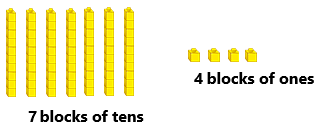We can write by using place value charts also.
Place value chart:
In Mathematics, place value charts help us to make sure that the digits are in the correct places. To identify the positional values of numbers accurately, first, write the digits in the place value chart and then write the numbers in the usual and the standard form.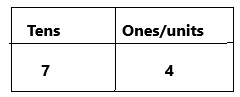Question 18.
H.O.T. Multi-Step A number has a digit less than 6 in the tens place and a digit greater than 6 in the ones place. Which of these could be the number?
(A) eighty-four
(B) sixty-six
(C) fifty-seven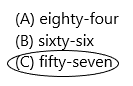Explanation:
In the question given, the digit less than 6 in the tens place and greater than 6 in one’s place. The digit in the tens place will be 5 because 5 is less than 6. And in one’s place, 7 will come because greater than 6. According to that, we need to write the answer.

Question 19.
Connect Marty’s team shirt has the number 19 on it. Which is another way to write 19?
(A) 1 + 9
(B) ninety
(C) 10 + 9Explanation:
The number present in Marty’s team shirt=19
This 19 can be written as an expanded form, word form.
An expanded form of 19 is 10+9. In the number 19, 1 is in the tens place and 9 is in one’s palce.

Question 20.
Representations There are fifty-seven balloons at the party. Which is another way to write fifty-seven?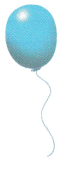(A) seventy-five
(B) 50 + 7
(C) 75Explanation:
50+7 is expanded form.
Expanded form: The expanded form of the numbers helps to determine the place value of each digit in the given number. It means that the expansion of numbers is based on the place value. The expanded form splits the number, and it represents the number in units, tens, hundreds and thousands form

Question 21.
Craig lives at 62 Kent Avenue. Which is another way to write 62?
(A) 20 + 6
(B) sixty-two
(C) 6 + 2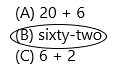Explanation:
Word form is writing the numerical/number as you would say it in words. Word form is one way in which the concept of numbers can be written. Other forms include standard and expanded forms. Word form involves expressing numbers using words rather than numerals. The word form for 2,010 is two thousand ten. The word form for 1,000,000 is one million. Likewise, 62 is given so it can be written as sixty-two.

Question 22.
TEXAS Test Prep Which is another way to write 92?
(A) 90 + 2
(B) twenty-four
(C) 4 + 2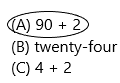Explanation:
I had written an expanded form.
Expanded form: The expanded form of the numbers helps to determine the place value of each digit in the given number. It means that the expansion of numbers is based on the place value. The expanded form splits the number, and it represents the number in units, tens, hundreds and thousands form. For example, the expanded form of the number 5679 is
5000+600+70+9.

TAKE HOME ACTIVITY • Write 20 + 6 on a sheet of paper. Have your child write the 2-digit number. Repeat for 4 tens 9 ones.
Explanation:
20+6 which is in the expanded form. And from this expanded form we have to write the two-digit number that can be written as 26.
This 26 can be written in word form as twenty-six.
In the number 26, the number 2 is in the tens place and 6 is in one’s place.
Likewise, we have to repeat for 4 tens and 9 ones.
This can be written in expanded form, word form, and pictorial representation.
Expanded form: The expanded form of the numbers helps to determine the place value of each digit in the given number. It means that the expansion of numbers is based on the place value. The expanded form splits the number, and it represents the number in units, tens, hundreds and thousands form.
The expanded form is 40+9
Word form: Word form is writing the numerical/number as you would say it in words.
The word form of 49 is forty-nine.
Pictorial representation:
We can have 4 blocks of tens and 9 blocks of ones.### Texas Go Math Grade 2 Lesson 1.1 Homework and Practice Answer Key

Write the number another way.

Question 1.
38
Explanation:
Word form is writing the numerical/number as you would say it in words. Word form is one way in which the concept of numbers can be written. Other forms include standard and expanded forms. Word form involves expressing numbers using words rather than numerals. The word form for 2,010 is two thousand ten. The word form for 1,000,000 is one million. Likewise, 38 is given so it can be written as thirty-eight.
Expanded form:
In expanded form 38 can be written as:
38= 30 + 8.

Question 2.
eighty-nine
Explanation:
The expanded form of the numbers helps to determine the place value of each digit in the given number. It means that the expansion of numbers is based on the place value. The expanded form splits the number, and it represents the number in units, tens, hundreds and thousands form. For example, the expanded form of the number 1327 is 1000+300+20+7.
Likewise, 89 can be written as 80+9.
8 is tens place and 9 is in one’s place.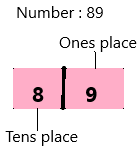Question 3.
30 + 7
Explanation:
Word form is writing the numerical/number as you would say it in words. Word form is one way in which the concept of numbers can be written. Other forms include standard and expanded forms. Word form involves expressing numbers using words rather than numerals. The word form for 2,010 is two thousand ten. The word form for 1,000,000 is one million. Likewise, 37 is given so it can be written as thirty-seven.

Question 4.
26
We can write in word form, expanded form, and pictorial representation.
Word form is writing the numerical/number as you would say it in words. Word form is one way in which the concept of numbers can be written. Other forms include standard and expanded forms. Word form involves expressing numbers using words rather than numerals. The word form for 2,010 is two thousand ten. The word form for 1,000,000 is one million.
Word form: Twenty-six.
Expanded form: The expanded form of the numbers helps to determine the place value of each digit in the given number. It means that the expansion of numbers is based on the place value. The expanded form splits the number, and it represents the number in units, tens, hundreds and thousands form. For example, the expanded form of the number 1572 is 1000+500+70+2.
The expanded form of 26 is 20+6. In the number 26, there are 2 tens and 6 ones.

Question 5.
fifteen
Explanation:
The expanded form of the numbers helps to determine the place value of each digit in the given number. It means that the expansion of numbers is based on the place value. The expanded form splits the number, and it represents the number in units, tens, hundreds and thousands form. For example, the expanded form of the number 1984 is 1000+900+80+4.
Likewise, the expanded form of 15 is 10+5. In the number 15, 1 is in the tens place and 5 is in one’s place.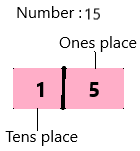Question 6.
60 + 2
Explanation:
We can write in word form, expanded form, and pictorial representation.
Word form is writing the numerical/number as you would say it in words. Word form is one way in which the concept of numbers can be written. Other forms include standard and expanded forms. Word form involves expressing numbers using words rather than numerals. The word form for 2,010 is two thousand ten. The word form for 1,000,000 is one million.
The word form of 62 is sixty-two.

Problem Solving

Circle the answer for each riddle.

Question 7.
Lee has a favorite number. The number has the digit 3 in the ones place and the digit 9 in the tens place. Which of these is another way to write this number?
(A) 30 + 9
(B) 90 + 3
(C) 3 + 9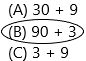Explanation:
Each number has a place value. It determines the value of that digit according to its position in the number. The value of a digit in a number increases as we move from left to right. The digits on the left have a lower place value than the digits on the right.
–  Use place value diagram to express the number:
– Number:93Question 8.
Multi-Step Rita’s locker code is a number that has a digit less than 3 in the tens place. It also has a digit greater than 7 in the ones place. Which of these could be the number?
(A) thirty-seven
(B) eighty-two
(C) twenty-eight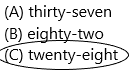Explanation:
In the question given greater than 7 in one’s place and less than 3 in tens place should write. According to the options above, option C is correct because there will be 8 in one’s place which is greater than 7 and 2 in tens place which is less than 3.

Lesson Check

Question 9.
Beth lives at 79 Main Street. Which is another way to write 79?
(A) 90 + 7
(B) seventy-nine
(C) 7 + 9
Explanation:
The second most common way we will see numbers in our life is word form.  It is important for students to know that ‘seventy-nine’ and 79 mean the same thing.  It is also important for us to know how to correctly write numbers.  Don’t forget the important hyphen starting at number 79!Question 10.
There are 48 muffins in a large basket. Which is another way to write 48?
(A) eighty-four
(B) 40 + 8
(C) 84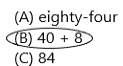Explanation:
Another important way of representing numbers is the expanded form which is the logical next step. In the expanded form, we break up a number according to its place value and expand it to show the value of each digit.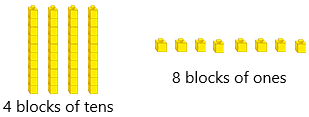Expanded form: 40 + 8
Each number has a place value. It determines the value of that digit according to its position in the number. The value of a digit in a number increases as we move from left to right. The digits on the left have a lower place value than the digits on the right.

Question 11.
Sue has eighty-seven buttons. Which is another way to write eighty-seven?
(A) 80 + 7
(B) seventy-eight
(C) 8 + 7Explanation:
Another important way of representing numbers is the expanded form which is the logical next step. In the expanded form, we break up a number according to its place value and expand it to show the value of each digit.Expanded form: 80 + 7
Each number has a place value. It determines the value of that digit according to its position in the number. The value of a digit in a number increases as we move from left to right. The digits on the left have a lower place value than the digits on the right.

Question 12.
Multi-Step Dan’s number has a digit greater than 5 in the ones place and a digit less than 5 in the tens place. Which could be the number on Dan’s shirt?
(A) 5 + 5
(B) forty-six
(C) 70 + 3